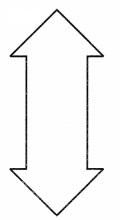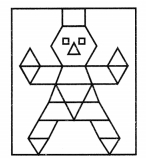## Grade 6 Maths Symmetry Multiple Choice Questions (MCQs)

1. A parallelogram has …………… lines of symmetry:
(a) 0
(b) 1
(c) 2
(d) 3

2. Which of the following alphabets has line symmetry?
(a) P
(b) Z
(c) A
(d) Q

3. How many lines of symmetries are there in an equilateral triangle?
(a) 1
(b) 2
(c) 3
(d) 4

4. How many lines of symmetries are there in an isosceles triangle?
(a) 1
(b) 2
(c) 3
(d) 4

5. How many lines of symmetries are there in a rhombus?
(a) 1
(b) 2
(c) 3
(d) 4

6. How many lines of symmetries are there in a square?
(a) 1
(b) 2
(c) 3
(d) 4

7. How many lines of symmetries are there in a regular pentagon?
(a) 1
(b) 2
(c) 3
(d) 4

8. How many lines of symmetries are there in a rectangle?
(a) 1
(b) 2
(c) 3
(d) 4

9. Find the number of lines of symmetry of the following figure:
(a) 1
(b) 2
(c) 3
(d) 410. Find the number of lines of symmetry of the following figure:
(a) 1
(b) 2
(c) 3
(d) 411. Find the number of lines of symmetry in a regular hexagon.
(a) 6
(b) 2
(c) 3
(d) 4

12. Find the number of lines of symmetry in the below left figure.
(a) 1
(b) 2
(c) 3
(d) 413. Find the number of lines of symmetry in the above right sided figure.
(a) 1
(b) 2
(c) 3
(d) none of these14. Find the number of lines of symmetry in the below left figure.
(a) 1
(b) 2
(c) 3
(d) 415. Find the number of lines of symmetry in the above right sided figure.
(a) 1
(b) 2
(c) 3
(d) 416. Find the number of lines of symmetry in a circle.
(a) 1
(b) 2
(c) 3
(d) none of these

17. Which of the following has no line of symmetry?
(a) S
(b) A
(c) U
(d) H

18. Which of the following has both horizontal as well as vertical line of symmetry?
(a) Z
(b) B
(c) P
(d) I

19. Find the number of lines of symmetry in a scalene triangle.
(a) 0
(b) 1
(c) 2
(d) 3

20. Which letter look the same after reflection when the mirror is placed vertically?
(a) Z
(b) P
(c) M
(d) N

### Class 6 Maths Symmetry Fill In The Blanks

1. The number of lines of symmetry in a picture of Taj Mahal is …………… .
2. The number of lines of symmetry in a rectangle and a square are ……………. (equal/unequal).
3. The digits having only two lines of symmetry are ………….. and …………… .
4. The number of capital letters of the English alphabets having no line of symmetry is ………….. .
5. The number of lines of symmetry in a regular hexagon is ………………. .

C. Open your geometry box. There are some drawing tools. Observe them and complete the following table:

 Number of the total Number of lines of symmetry 1. The Ruler 2. The Divider 3. The Compasses 4. The Protractor 5. Triangular piece with two equal sides 6. Triangular piece with unequal sides

### Class 6 Maths Symmetry Match the following

 Shape Number of lines of symmetry 1. Square a. 5 2. Kite b. 4 3. Equilateral triangle c. 3 4. Rectangle d. 2 5. Regular hexagon e. 1 6. Scalene triangle f. 0 7. Circle g. 6 8. Regular pentagon h. Infinitely many

### Class 6 Maths Symmetry Short Answer Type Questions

1. Write all the capital letters of the English alphabets which have more than one lines of symmetry.
2. Draw the following shapes and find the number of lines of symmetry
(a) Equilateral triangle
(b) Rectangle
(c) Square
(d) Parallelogram
3. On Squared paper, sketch the following:
(a) A hexagon with exactly two lines of symmetry.
(b) A triangle that has no line of symmetry.### Class 6 Maths Symmetry Long Answer Type Questions

1. On Squared paper, sketch the following:
(a) A triangle with a horizontal line of symmetry but no vertical line of symmetry.
(b) A quadrilateral with a horizontal line of symmetry but no vertical line of symmetry.2. Write the letters of the word ‘MATHEMATICS’ which have no line of symmetry.
3. Write the number of lines of symmetry in each letter of the word ‘SYMMETRY’.